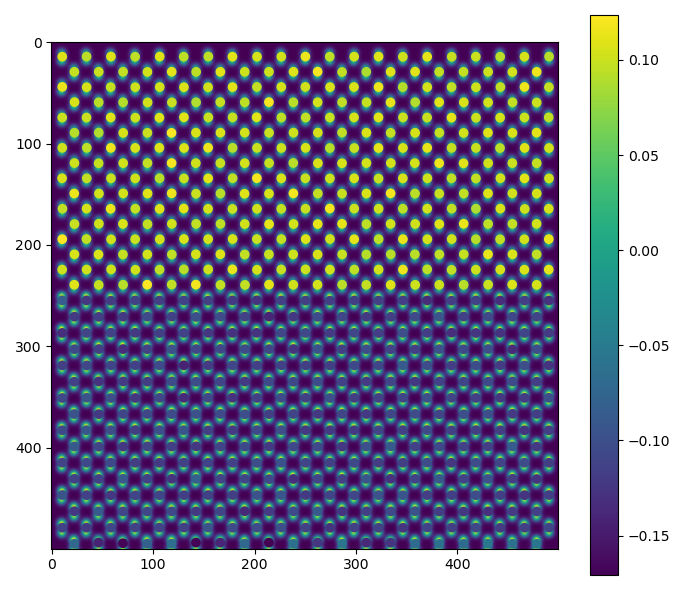# Dumbbell lattice¶

There is also a notebook in the Atomap-demos repository: https://gitlab.com/atomap/atomap_demos/blob/release/dumbbell_example_notebook/dumbbell_example.ipynb

Analysis of atomic resolution images can be tricky if the atoms are too close together, leading to overlap. For these images, several atomic columns must be fitted simultaneously. However, fitting many 2-D Gaussians over a large at the same time will be significantly slower than fitting a couple. So in these cases, only the atoms which overlap should be fitted simultaneously.

A very common example of this is dumbbells, often seen in zincblende structures such as Si or GaAs. Processing and analysing these require some specialized functions, which will be shown here. To do this, we’ll use a dataset emulating a zincblende heterostructure.

```>>> import atomap.api as am
>>> s = am.dummy_data.get_dumbbell_heterostructure_signal()
>>> s.plot()
```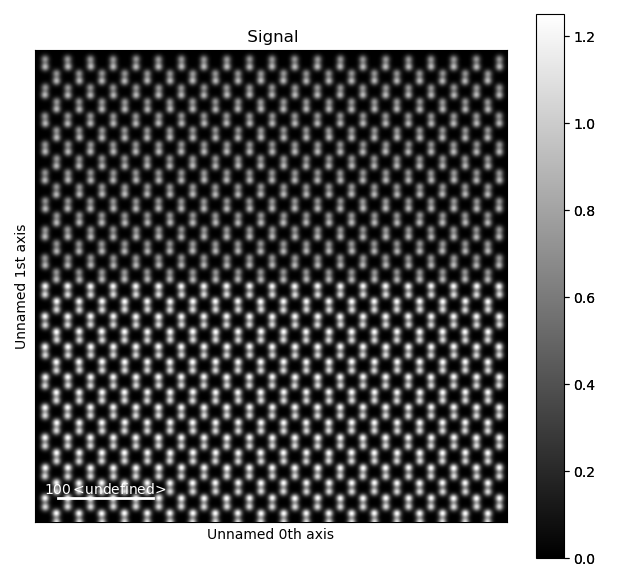## Dumbbell vector¶

The first step is finding the “dumbbell vector”, which is the distance and direction between the atoms in the dumbbells. We do this by getting all the atomic positions, firstly by finding an appropriate feature separation. As the atoms in the dumbbells are typically pretty close together, we use a low `separation_range` between 2 and 6.

```>>> s_peaks = am.get_feature_separation(s, separation_range=(2, 6), show_progressbar=False)
>>> s_peaks.plot()
```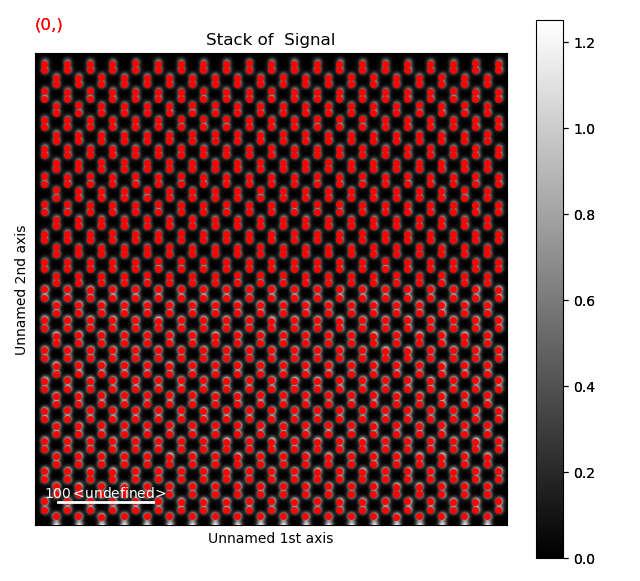The first separation, 2, seems to work well here. Use `get_atom_positions()` to get an array with all of these atomic positions.

```>>> atom_positions = am.get_atom_positions(s, separation=2)
```

To find the dumbbell vector, these atom positions are passed to the `find_dumbbell_vector()` function:

```>>> import atomap.initial_position_finding as ipf
>>> dumbbell_vector = ipf.find_dumbbell_vector(atom_positions)
```

## Making the dumbbell lattice¶

Then the dumbbells themselves has to be found, by finding one atom in each of the dumbbells. As these are further apart, we use a higher `separation_range` between 5 and 20.

```>>> s_peaks = am.get_feature_separation(s, separation_range=(5, 20), show_progressbar=False)
>>> s_peaks.plot()
```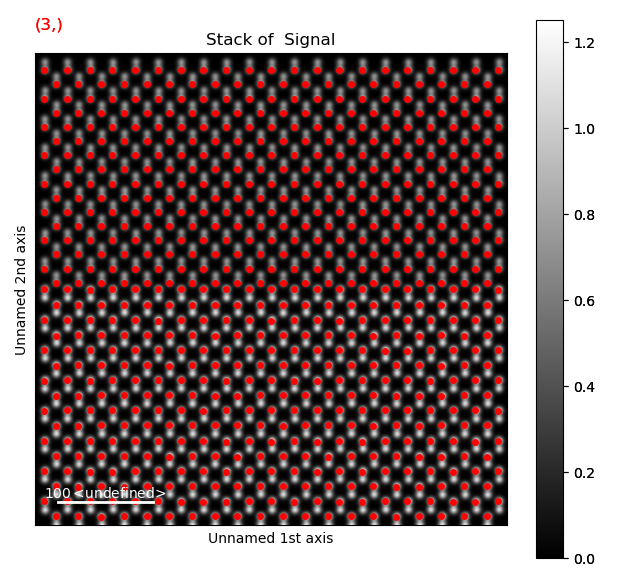Here, the third separation, 8, seems to work well. Use this get the `dumbbell_positions`.

```>>> dumbbell_positions = am.get_atom_positions(s, separation=8)
```

The `dumbbell_vector` and `dumbbell_positions` are then used to make a dumbbell_lattice, with the `make_atom_lattice_dumbbell_structure()` function:

```>>> dumbbell_lattice = ipf.make_atom_lattice_dumbbell_structure(s, dumbbell_positions, dumbbell_vector)
>>> dumbbell_lattice.plot()
```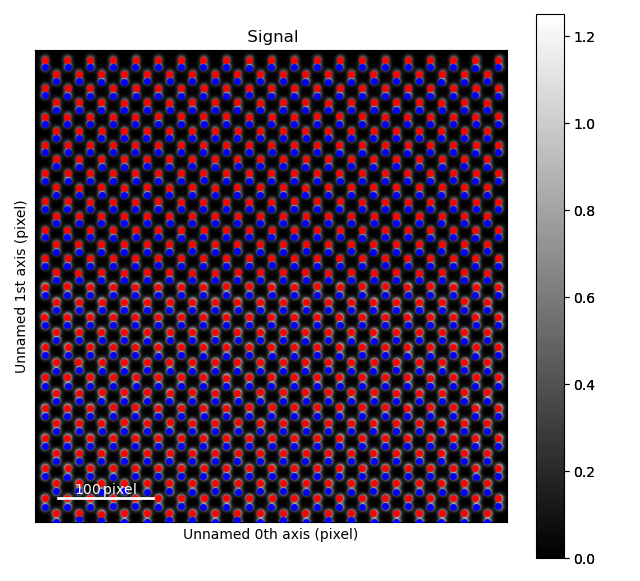Due to the atom columns being too close together, we can’t use the standard `refine` methods, but rather have to use a special one which fits both atoms in the dumbbell at the same time: `atomap.atom_lattice.Dumbbell_Lattice.refine_position_gaussian()`.

```>>> dumbbell_lattice.refine_position_gaussian()
```

## Analysing the image¶

There are several ways to analyse the image using the `Dumbbell_Lattice` object. Many of these are shared with the `Atom_Lattice` class, examples of these are seen in Analysing atom lattices.

A relevant one for a heterostructure is the atom column intensity.

```>>> i_points, i_record, p_record = dumbbell_lattice.integrate_column_intensity()
>>> i_record.plot()
```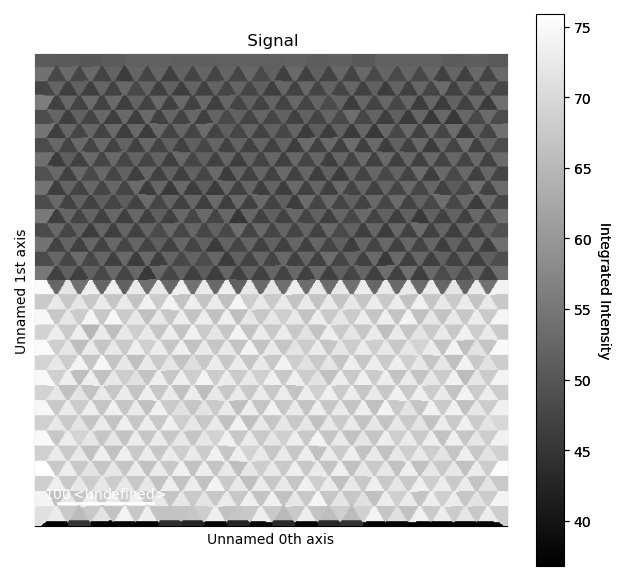Or out-of-plane lattice plane distances

```>>> sublattice0 = dumbbell_lattice.sublattice_list
>>> sublattice0.construct_zone_axes()
>>> out_of_plane_direction = sublattice0.zones_axis_average_distances
>>> interface_plane = sublattice0.atom_planes_by_zone_vector[out_of_plane_direction]
>>> s_out_of_plane_map = sublattice0.get_monolayer_distance_map([out_of_plane_direction, ], atom_plane_list=[interface_plane])
>>> s_out_of_plane_map.plot()
```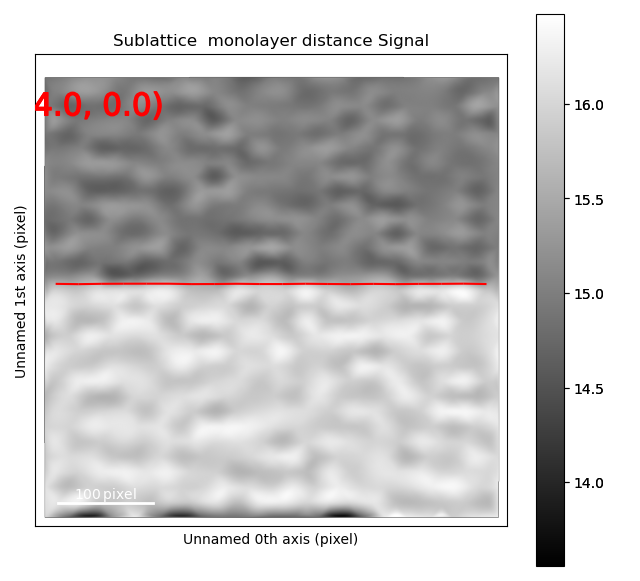```>>> s_out_of_plane_line_profile = sublattice0.get_monolayer_distance_line_profile(out_of_plane_direction, atom_plane=interface_plane)
>>> s_out_of_plane_line_profile.plot()
```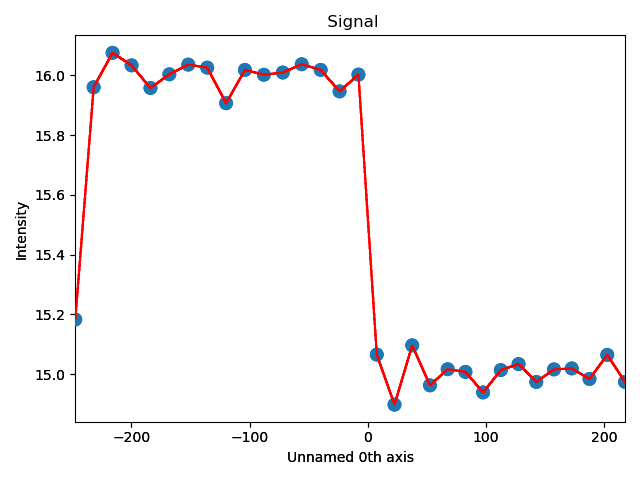There are also some specific for the `Dumbbell_Lattice` object:

1. `dumbbell_x`, the x-position of the dumbbell, which is defined as the mean x-position of the two atoms in the dumbbell
2. `dumbbell_y`, the y-position of the dumbbell, which is defined as the mean y-position of the two atoms in the dumbbell
3. `dumbbell_distance`, distance between the atoms in each dumbbell
4. `dumbbell_angle`, angle between the atoms in each dumbbell, in relation to the horizontal axis
5. `get_dumbbell_intensity_difference`, intensity difference between the two atoms in the dumbbell

These can be visualized using various plot functions. For `dumbbell_distance`:

```>>> fig = dumbbell_lattice.plot_dumbbell_distance()
```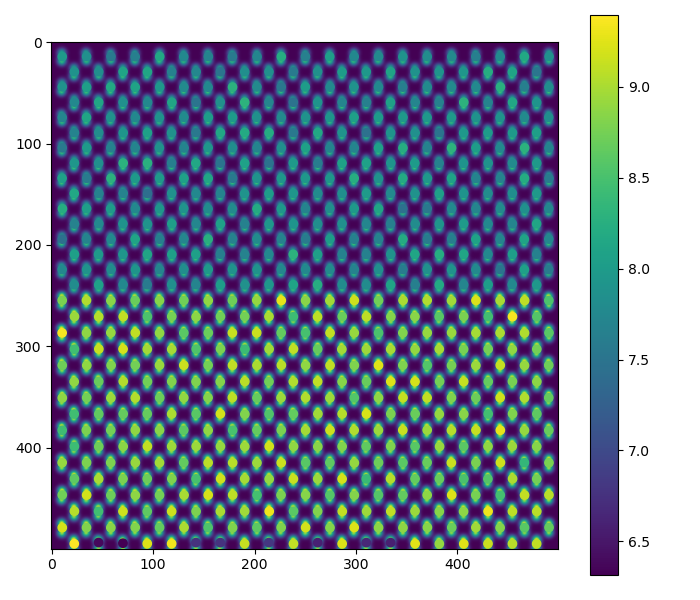For `dumbbell_angle`:

```>>> fig = dumbbell_lattice.plot_dumbbell_angle()
```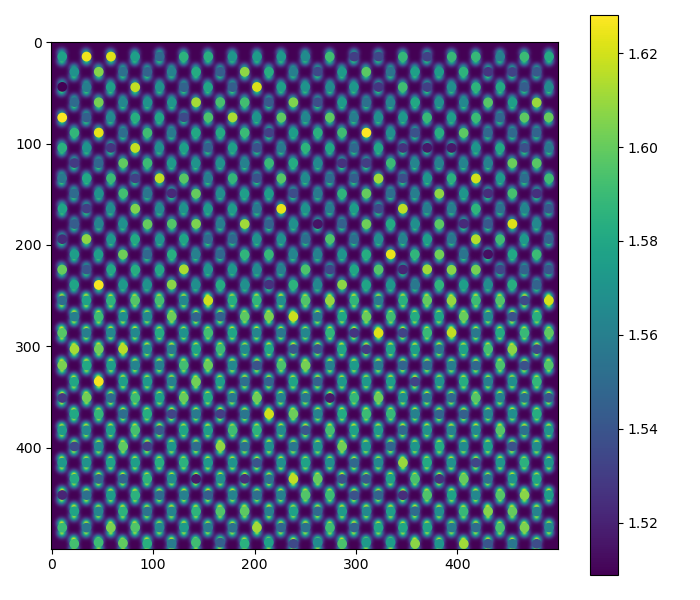For the dumbbell intensity difference:

```>>> fig = dumbbell_lattice.plot_dumbbell_intensity_difference()
```